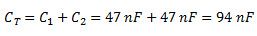# Capacitors In Series and Parallel Combinations

## Capacitors in Series and Parallel Combinations

Capacitors are one of the standard components in electronic and electrical circuits. However, complicated combinations of capacitors mostly occur in practical circuits.

It is, therefore, useful to have a set of rules for finding the equivalent capacitance of some general capacitors arrangements.

The equivalent capacitance of any complicated arrangement can be determined by the repeated application of two simple rules and these rules are related to capacitors connected in series and in parallel.

### Capacitors in Series

Capacitors are said to be connected in series, when they are effectively daisy chained together in a single line.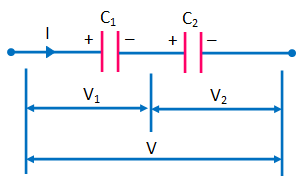Consider two capacitors connected in series: i.e. in a line such that the positive plate of one is attached to the negative plate of the other as shown in the fig. above.

In fact, let us suppose that the positive plate of capacitor 1 is connected to the input wire, the negative plate of capacitor 1 is connected to the positive plate of capacitor 2, and the negative plate of capacitor 2 is connected to the output wire.

Now the question arises, what is the equivalent capacitance between the input and output wires?

In this connection, it is important to realize that the charge Q stored in the two capacitors is same.

This can be explained as follows :

Let us consider the internal plates i.e., the negative plate of capacitor 1, and the positive plate of capacitor 2.

These plates are physically disconnected from the rest of the circuit, so the total charge on them must remain constant.

Assuming, that these plates carry zero charge when zero potential difference is applied across the two capacitors, it follows that in the presence of a non-zero potential difference the charge Q on the positive plate of capacitor 2 must be balanced by an equal and opposite charge -Q on the negative plate of capacitor 1.

Since the negative plate of capacitor 1 carries a charge -Q , the positive plate of capacitor 2 carries a charge + Q  to balance it.

As a result, both capacitors possess the same stored charge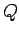.

The potential drops,and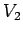, across the two capacitors different.

However, the sum of these drops equals the total potential dropapplied across the input and output wires:

i.e.The equivalent capacitance of the pair of capacitors is again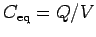. Thus,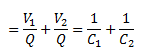Hence,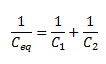Hence, we can conclude that :

The reciprocal of the equivalent capacitance of two capacitors connected in series is the sum of the reciprocals of the individual capacitances.

Forcapacitors connected in series, the equivalent capacitance equation can be  generalized to :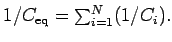### Example

Find the overall capacitance and the individual rms voltage drops across the two capacitors each with 47 nF ,in series when connected to a 12V a.c. supply.

Solution :

Total Capacitance,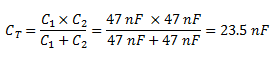Voltage drop across the two identical 47 nF capacitors,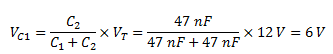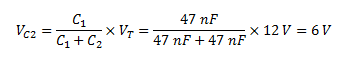### Capacitors in Parallel

Capacitors are said to be connected in parallel when both of their terminals are respectively connected to each terminal of the other capacitor or capacitors.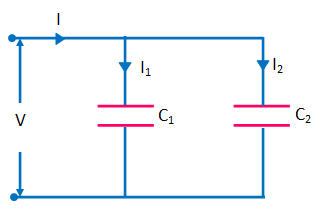Consider two capacitors connected in parallel: i.e., with the positively charged plates connected to a common input wire, and the negatively charged plates attached to a common output wire as shown in the above fig.

What is the equivalent capacitance between the input and output wires?

In this case, the potential differenceacross the two capacitors is the same, and is equal to the potential differnce between the input and output wires.

However, the total stored charge Q is divided between the two capacitors, since it must distribute itself such that the voltage across them is same.

Since, the capacitors may have different capacitances,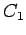and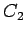, the charges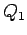and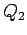may also be different.

The equivalent capacitance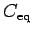of the pair of capacitors is simply the ratio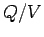,

where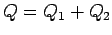is the total stored charge.

It follows that :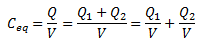Hence,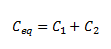In general for we can say that :

The equivalent capacitance of two capacitors connected in parallel is the sum of the individual capacitances.

Forcapacitors connected in parallel, the equation for equivalent capacitance can be generalizes to :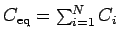Example :

Calculate the combined capacitance  of the following capacitors each with a capacitance of 47 nF, when they are connected together in a parallel combination .

Solution :

Total Capacitance,